# Practical guide to implement machine learning with CARET package in R (with practice problem)

## Introduction

One of the biggest challenge beginners in machine learning face is which algorithms to learn and focus on. In case of R, the problem gets accentuated by the fact that various algorithms would have different syntax, different parameters to tune and different requirements on the data format. This could be too much for a beginner.

So, then how do you transform from a beginner to a data scientist building hundreds of models and stacking them together? There certainly isn’t any shortcut but what I’ll tell you today will make you capable of applying hundreds of machine learning models without having to:

• remember the different package names for each algorithm.
• syntax of applying each algorithm.
• parameters to tune for each algorithm.

All this has been made possible by the years of effort that have gone behind CARET ( Classification And Regression Training) which is possibly the biggest project in R. This package alone is all you need to know for solve almost any supervised machine learning problem. It provides a uniform interface to several machine learning algorithms and standardizes various other tasks such as Data splitting, Pre-processing, Feature selection, Variable importance estimation, etc.

To get an in-depth overview of various functionalities provided by Caret, you can refer to this article.

Today, we’ll work on the Loan Prediction problem-III to show you the power of Caret package.

P.S. While caret definitely simplifies the job to a degree, it can not take away the hard work and practice you need to put in to become a master at machine learning.

## Table of Contents

1. Getting started
2. Pre-processing using Caret
3. Splitting the data using Caret
4. Feature selection using Caret
5. Training models using Caret
6. Parameter tuning using Caret
7. Variable importance estimation using Caret
8. Making predictions using Caret

## 1. Getting started

To put in simple words, Caret is essentially a wrapper for 200+ machine learning algorithms. Additionally, it provides several features which makes it a one stop solution for all the modeling needs for supervised machine learning problems.

Caret tries not to load all the packages it depends upon at the start. Instead, it loads them only when the packages are needed. But it does assume that you already have all the algorithms installed on your system.

To install Caret on your system, use the following command. Heads up: It might take some time:

``> install.packages("caret", dependencies = c("Depends", "Suggests"))``

Now, let’s get started using caret package on Loan Prediction 3 problem:

````#Loading caret package`
`library("caret")`

`#Loading training data`
`train<-read.csv("train_u6lujuX_CVtuZ9i.csv",stringsAsFactors = T)`

`#Looking at the structure of caret package.`
`str(train)`
`#'data.frame':         614 obs. of  13 variables:`
`#\$ Loan_ID          : Factor w/ 614 levels "LP001002","LP001003",..: 1 2 3 4 5 6 7 8 9 #10 ..`
`#\$ Gender           : Factor w/ 3 levels "","Female","Male": 3 3 3 3 3 3 3 3 3 3 ...`
`#\$ Married          : Factor w/ 3 levels "","No","Yes": 2 3 3 3 2 3 3 3 3 3 ...`
`#\$ Dependents       : Factor w/ 5 levels "","0","1","2",..: 2 3 2 2 2 4 2 5 4 3 ...`
`#\$ Education        : Factor w/ 2 levels "Graduate","Not Graduate": 1 1 1 2 1 1 2 1 1 #1 ...`
`#\$ Self_Employed    : Factor w/ 3 levels "","No","Yes": 2 2 3 2 2 3 2 2 2 2 ...`
`#\$ ApplicantIncome  : int  5849 4583 3000 2583 6000 5417 2333 3036 4006 12841 #...`
`#\$ CoapplicantIncome: num  0 1508 0 2358 0 ...`
`#\$ LoanAmount       : int  NA 128 66 120 141 267 95 158 168 349 ...`
`#\$ Loan_Amount_Term : int  360 360 360 360 360 360 360 360 360 360 ...`
`#\$ Credit_History   : int  1 1 1 1 1 1 1 0 1 1 ...`
`#\$ Property_Area    : Factor w/ 3 levels "Rural","Semiurban",..: 3 1 3 3 3 3 3 2 3 2 #...`
`#\$ Loan_Status      : Factor w/ 2 levels "N","Y": 2 1 2 2 2 2 2 1 2 1 ...````

In this problem, we have to predict the Loan Status of a person based on his/ her profile.

## 2. Pre-processing using Caret

We need to pre-process our data before we can use it for modeling. Let’s check if the data has any missing values:

````sum(is.na(train))`
`# 86````

Next, let us use Caret to impute these missing values using KNN algorithm. We will predict these missing values based on other attributes for that row. Also, we’ll scale and center the numerical data by using the convenient preprocess() in Caret.

````#Imputing missing values using KNN.Also centering and scaling numerical columns`
`preProcValues <- preProcess(train, method = c("knnImpute","center","scale"))`

`library('RANN')`
`train_processed <- predict(preProcValues, train)`
`sum(is.na(train_processed))`
`# 0````

It is also very easy to use one hot encoding in Caret to create dummy variables for each level of a categorical variable. But first, we’ll convert the dependent variable to numerical.

````#Converting outcome variable to numeric`
`train_processed\$Loan_Status<-ifelse(train_processed\$Loan_Status=='N',0,1)`

`id<-train_processed\$Loan_ID`
`train_processed\$Loan_ID<-NULL`

`#Checking the structure of processed train file`
`str(train_processed)`
`#'data.frame':         614 obs. of  12 variables:`
`#\$ Gender           : Factor w/ 3 levels "","Female","Male": 3 3 3 3 3 3 3 3 3 3 ...`
`#\$ Married          : Factor w/ 3 levels "","No","Yes": 2 3 3 3 2 3 3 3 3 3 ...`
`#\$ Dependents       : Factor w/ 5 levels "","0","1","2",..: 2 3 2 2 2 4 2 5 4 3 ...`
`#\$ Education        : Factor w/ 2 levels "Graduate","Not Graduate": 1 1 1 2 1 1 2 1 1 #1 ...`
`#\$ Self_Employed    : Factor w/ 3 levels "","No","Yes": 2 2 3 2 2 3 2 2 2 2 ...`
`#\$ ApplicantIncome  : num  0.0729 -0.1343 -0.3934 -0.4617 0.0976 ...`
`#\$ CoapplicantIncome: num  -0.554 -0.0387 -0.554 0.2518 -0.554 ...`
`#\$ LoanAmount       : num  0.0162 -0.2151 -0.9395 -0.3086 -0.0632 ...`
`#\$ Loan_Amount_Term : num  0.276 0.276 0.276 0.276 0.276 ...`
`#\$ Credit_History   : num  0.432 0.432 0.432 0.432 0.432 ...`
`#\$ Property_Area    : Factor w/ 3 levels "Rural","Semiurban",..: 3 1 3 3 3 3 3 2 3 2 #...`
`#\$ Loan_Status      : num  1 0 1 1 1 1 1 0 1 0 ...````

Now, creating dummy variables using one hot encoding:

````#Converting every categorical variable to numerical using dummy variables`
`dmy <- dummyVars(" ~ .", data = train_processed,fullRank = T)`
`train_transformed <- data.frame(predict(dmy, newdata = train_processed))`

`#Checking the structure of transformed train file`
`str(train_transformed)`
`#'data.frame':         614 obs. of  19 variables:`
`#\$ Gender.Female          : num  0 0 0 0 0 0 0 0 0 0 ...`
`#\$ Gender.Male            : num  1 1 1 1 1 1 1 1 1 1 ...`
`#\$ Married.No             : num  1 0 0 0 1 0 0 0 0 0 ...`
`#\$ Married.Yes            : num  0 1 1 1 0 1 1 1 1 1 ...`
`#\$ Dependents.0           : num  1 0 1 1 1 0 1 0 0 0 ...`
`#\$ Dependents.1           : num  0 1 0 0 0 0 0 0 0 1 ...`
`#\$ Dependents.2           : num  0 0 0 0 0 1 0 0 1 0 ...`
`#\$ Dependents.3.          : num  0 0 0 0 0 0 0 1 0 0 ...`
`#\$ Education.Not.Graduate : num  0 0 0 1 0 0 1 0 0 0 ...`
`#\$ Self_Employed.No       : num  1 1 0 1 1 0 1 1 1 1 ...`
`#\$ Self_Employed.Yes      : num  0 0 1 0 0 1 0 0 0 0 ...`
`#\$ ApplicantIncome        : num  0.0729 -0.1343 -0.3934 -0.4617 0.0976 ...`
`#\$ CoapplicantIncome      : num  -0.554 -0.0387 -0.554 0.2518 -0.554 ...`
`#\$ LoanAmount             : num  0.0162 -0.2151 -0.9395 -0.3086 -0.0632 ...`
`#\$ Loan_Amount_Term       : num  0.276 0.276 0.276 0.276 0.276 ...`
`#\$ Credit_History         : num  0.432 0.432 0.432 0.432 0.432 ...`
`#\$ Property_Area.Semiurban: num  0 0 0 0 0 0 0 1 0 1 ...`
`#\$ Property_Area.Urban    : num  1 0 1 1 1 1 1 0 1 0 ...`
`#\$ Loan_Status            : num  1 0 1 1 1 1 1 0 1 0 ...`

`#Converting the dependent variable back to categorical`
`train_transformed\$Loan_Status<-as.factor(train_transformed\$Loan_Status)````

Here, “fullrank=T” will create only (n-1) columns for a categorical column with n different levels. This works well particularly for the representing categorical predictors like gender, married, etc. where we only have two levels: Male/Female, Yes/No, etc. because 0 can be used to represent one class while 1 represents the other class in same column.

## 3. Splitting data using caret

We’ll be creating a cross-validation set from the training set to evaluate our model against. It is important to rely more on the cross-validation set for the actual evaluation of your model otherwise you might end up overfitting the public leaderboard.

We’ll use createDataPartition() to split our training data into two sets : 75% and 25%. Since, our outcome variable is categorical in nature, this function will make sure that the distribution of outcome variable classes will be similar in both the sets.

````#Spliting training set into two parts based on outcome: 75% and 25%`
`index <- createDataPartition(train_transformed\$Loan_Status, p=0.75, list=FALSE)`
`trainSet <- train_transformed[ index,]`
`testSet <- train_transformed[-index,]`

`#Checking the structure of trainSet`
`str(trainSet)`
`#'data.frame':         461 obs. of  19 variables:`
`#\$ Gender.Female          : num  0 0 0 0 0 0 0 0 0 0 ...`
`#\$ Gender.Male            : num  1 1 1 1 1 1 1 1 1 1 ...`
`#\$ Married.No             : num  1 0 0 0 1 0 0 0 0 0 ...`
`#\$ Married.Yes            : num  0 1 1 1 0 1 1 1 1 1 ...`
`#\$ Dependents.0           : num  1 0 1 1 1 0 1 0 0 0 ...`
`#\$ Dependents.1           : num  0 1 0 0 0 0 0 0 1 0 ...`
`#\$ Dependents.2           : num  0 0 0 0 0 1 0 0 0 1 ...`
`#\$ Dependents.3.          : num  0 0 0 0 0 0 0 1 0 0 ...`
`#\$ Education.Not.Graduate : num  0 0 0 1 0 0 1 0 0 0 ...`
`#\$ Self_Employed.No       : num  1 1 0 1 1 0 1 1 1 1 ...`
`#\$ Self_Employed.Yes      : num  0 0 1 0 0 1 0 0 0 0 ...`
`#\$ ApplicantIncome        : num  0.0729 -0.1343 -0.3934 -0.4617 0.0976 ...`
`#\$ CoapplicantIncome      : num  -0.554 -0.0387 -0.554 0.2518 -0.554 ...`
`#\$ LoanAmount             : num  0.0162 -0.2151 -0.9395 -0.3086 -0.0632 ...`
`#\$ Loan_Amount_Term       : num  0.276 0.276 0.276 0.276 0.276 ...`
`#\$ Credit_History         : num  0.432 0.432 0.432 0.432 0.432 ...`
`#\$ Property_Area.Semiurban: num  0 0 0 0 0 0 0 1 1 0 ...`
`#\$ Property_Area.Urban    : num  1 0 1 1 1 1 1 0 0 1 ...`
`#\$ Loan_Status            : Factor w/ 2 levels "0","1": 2 1 2 2 2 2 2 1 1 2 ...````

## 4. Feature selection using Caret

Feature selection is an extremely crucial part of modeling. To understand the importance of feature selection and various techniques used for feature selection, I strongly recommend that you to go through my previous article. For now, we’ll be using Recursive Feature elimination which is a wrapper method to find the best subset of features to use for modeling.

```#Feature selection using rfe in caret
`control <- rfeControl(functions = rfFuncs,`
`                   method = "repeatedcv",`
`                   repeats = 3,`
`                   verbose = FALSE)`
`outcomeName<-'Loan_Status'`
`predictors<-names(trainSet)[!names(trainSet) %in% outcomeName]`
`Loan_Pred_Profile <- rfe(trainSet[,predictors], trainSet[,outcomeName],`
`                      rfeControl = control)`
`Loan_Pred_Profile`
`#Recursive feature selection`
`#Outer resampling method: Cross-Validated (10 fold, repeated 3 times)`
`#Resampling performance over subset size:`
`#  Variables Accuracy  Kappa AccuracySD KappaSD Selected`
`#4   0.7737 0.4127    0.03707 0.09962        `
`#8   0.7874 0.4317    0.03833 0.11168        `
`#16   0.7903 0.4527    0.04159 0.11526        `
`#18   0.7882 0.4431    0.03615 0.10812        `
`#The top 5 variables (out of 16):`
`#  Credit_History, LoanAmount, Loan_Amount_Term, ApplicantIncome, CoapplicantIncome`
`#Taking only the top 5 predictors`
`predictors<-c("Credit_History", "LoanAmount", "Loan_Amount_Term", "ApplicantIncome", "CoapplicantIncome")````

## 5. Training models using Caret

This is probably the part where Caret stands out from any other available package. It provides the ability for implementing 200+ machine learning algorithms using consistent syntax. To get a list of all the algorithms that Caret supports, you can use:

```names(getModelInfo())
`# "ada"                 "AdaBag"              "AdaBoost.M1"         "adaboost"          `
`# "amdai"               "ANFIS"               "avNNet"              "awnb"              `
`# "awtan"               "bag"                 "bagEarth"            "bagEarthGCV"       `
`# "bagFDA"              "bagFDAGCV"           "bam"                 "bartMachine"       `
`# "bayesglm"            "bdk"                 "binda"               "blackboost"        `
`# "blasso"              "blassoAveraged"      "Boruta"              "bridge"             `
`#….`
`# "svmBoundrangeString" "svmExpoString"       "svmLinear"           "svmLinear2"        `
`# "svmLinear3"          "svmLinearWeights"    "svmLinearWeights2"   "svmPoly"           `
`# "svmRadial"           "svmRadialCost"       "svmRadialSigma"      "svmRadialWeights"  `
`# "svmSpectrumString"   "tan"                 "tanSearch"           "treebag"           `
`# "vbmpRadial"          "vglmAdjCat"          "vglmContRatio"       "vglmCumulative"    `
`# "widekernelpls"       "WM"                  "wsrf"                "xgbLinear"         `
`# "xgbTree"             "xyf"````

To get more details of any model, you can refer here.

We can simply apply a large number of algorithms with similar syntax. For example, to apply, GBM, Random forest, Neural net and Logistic regression :

````model_gbm<-train(trainSet[,predictors],trainSet[,outcomeName],method='gbm')`
`model_rf<-train(trainSet[,predictors],trainSet[,outcomeName],method='rf')`
`model_nnet<-train(trainSet[,predictors],trainSet[,outcomeName],method='nnet')`
`model_glm<-train(trainSet[,predictors],trainSet[,outcomeName],method='glm')````

You can proceed further tune the parameters in all these algorithms using the parameter tuning techniques.

## 6. Parameter tuning using Caret

It’s extremely easy to tune parameters using Caret. Typically, parameter tuning in Caret is done as below: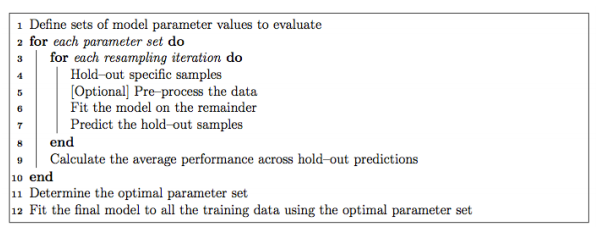It is possible to customize almost every step in the tuning process. The resampling technique used for evaluating the performance of the model using a set of parameters in Caret by default is bootstrap, but it provides alternatives for using k-fold, repeated k-fold as well as Leave-one-out cross validation (LOOCV) which can be specified using trainControl(). In this example, we’ll be using 5-Fold cross-validation repeated 5 times.

````fitControl <- trainControl(`
`  method = "repeatedcv",`
`  number = 5,`
`  repeats = 5)````

If the search space for parameters is not defined, Caret will use 3 random values of each tunable parameter and use the cross-validation results to find the best set of parameters for that algorithm. Otherwise, there are two more ways to tune parameters:

### 6.1.Using tuneGrid

To find the parameters of a model that can be tuned, you can use

````modelLookup(model='gbm')`

`#model         parameter                   label forReg forClass probModel`
`#1   `gbm`           n.trees   # Boosting Iterations   TRUE     TRUE      TRUE`
`#2   `gbm` interaction.depth          Max Tree Depth   TRUE     TRUE      TRUE`
`#3   `gbm`         shrinkage               Shrinkage   TRUE     TRUE      TRUE`
`#4   `gbm`    n.minobsinnode Min. Terminal Node Size   TRUE     TRUE      TRUE`
`#using grid search`

`#Creating grid`
`grid <- expand.grid(n.trees=c(10,20,50,100,500,1000),shrinkage=c(0.01,0.05,0.1,0.5),n.minobsinnode = c(3,5,10),interaction.depth=c(1,5,10))`

`# training the model`
`model_gbm<-train(trainSet[,predictors],trainSet[,outcomeName],method='gbm',trControl=fitControl,tuneGrid=grid)`

`# summarizing the model`
`print(model_gbm)`

`#Stochastic Gradient Boosting`
`#461 samples`
`#5 predictor`
`#2 classes: '0', '1'`

#`No pre-processing`
`#Resampling: Cross-Validated (5 fold, repeated 5 times)`
`#Summary of sample sizes: 368, 370, 369, 369, 368, 369, ...`
`#Resampling results across tuning parameters:`

`#  shrinkage  interaction.depth  n.minobsinnode  n.trees  Accuracy   Kappa   `
`#0.01        1                  3                10     0.6876416  0.000000`0
#`0.01        1                  3                20     0.6876416  0.0000000`
`#0.01        1                  3                50     0.7982345  0.4423609`
`#0.01        1                  3               100     0.7952190  0.4364383`
`#0.01        1                  3               500     0.7904882  0.4342300`
`#0.01        1                  3              1000     0.7913627  0.4421230`
`#0.01        1                  5                10     0.6876416  0.0000000`
`#0.01        1                  5                20     0.6876416  0.0000000`
`#0.01        1                  5                50     0.7982345  0.4423609`
`#0.01        1                  5               100     0.7943635  0.4351912`
`#0.01        1                  5               500     0.7930783  0.4411348`
`#0.01        1                  5              1000     0.7913720  0.4417463`
`#0.01        1                 10                10     0.6876416  0.0000000`
`#0.01        1                 10                20     0.6876416  0.0000000`
`#0.01        1                 10                50     0.7982345  0.4423609`
`#0.01        1                 10               100     0.7943635  0.4351912`
`#0.01        1                 10               500     0.7939525  0.4426503`
`#0.01        1                 10              1000     0.7948362  0.4476742`
`#0.01        5                  3                10     0.6876416  0.0000000`
`#0.01        5                  3                20     0.6876416  0.0000000`
`#0.01        5                  3                50     0.7960556  0.4349571`
`#0.01        5                  3               100     0.7934987  0.4345481`
`#0.01        5                  3               500     0.7775055  0.4147204`
`#...`
`#0.50        5                 10               100     0.7045617  0.2834696`
`#0.50        5                 10               500     0.6924480  0.2650477`
`#0.50        5                 10              1000     0.7115234  0.3050953`
`#0.50       10                  3                10     0.7389117  0.3681917`
`#0.50       10                  3                20     0.7228519  0.3317001`
`#0.50       10                  3                50     0.7180833  0.3159445`
`#0.50       10                  3               100     0.7172417  0.3189655`
`#0.50       10                  3               500     0.7058472  0.3098146`
`#0.50       10                  3              1000     0.7001852  0.2967784`
`#0.50       10                  5                10     0.7266895  0.3378430`
`#0.50       10                  5                20     0.7154746  0.3197905`
`#0.50       10                  5                50     0.7063535  0.2984819`
`#0.50       10                  5               100     0.7151012  0.3141440`
`#0.50       10                  5               500     0.7108516  0.3146822`
`#0.50       10                  5              1000     0.7147320  0.3225373`
`#0.50       10                 10                10     0.7314871  0.3327504`
`#0.50       10                 10                20     0.7150814  0.3081869`
`#0.50       10                 10                50     0.6993723  0.2815981`
`#0.50       10                 10               100     0.6977416  0.2719140`
`#0.50       10                 10               500     0.7037864  0.2854748`
`#0.50       10                 10              1000     0.6995610  0.2869718````

Accuracy was used to select the optimal model using  the largest value.

The final values used for the model were n.trees = 10, interaction.depth = 1, shrinkage = 0.05 and n.minobsinnode = 3

`plot(model_gbm)`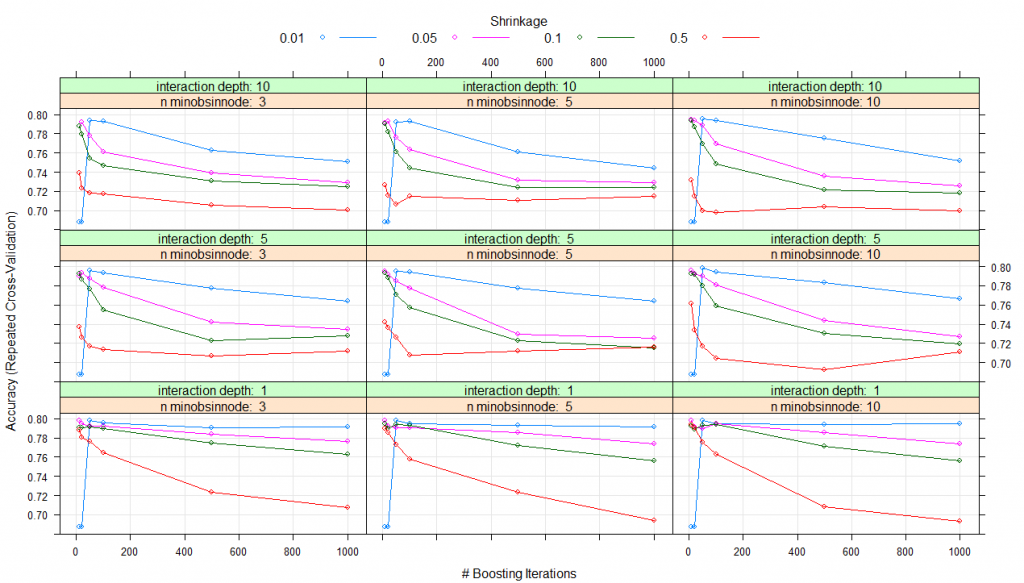Thus, for all the parameter combinations that you listed in expand.grid(), a model will be created and tested using cross-validation. The set of parameters with the best cross-validation performance will be used to create the final model which you get at the end.

### 6.2. Using tuneLength

Instead, of specifying the exact values for each parameter for tuning we can simply ask it to use any number of possible values for each tuning parameter through tuneLength. Let’s try an example using tuneLength=10.

````#using tune length`
`model_gbm<-train(trainSet[,predictors],trainSet[,outcomeName],method='gbm',trControl=fitControl,tuneLength=10)`
`print(model_gbm)`

`#Stochastic Gradient Boosting`
`#461 samples`
`#5 predictor`
`#2 classes: '0', '1'`

#`No pre-processing`
`#Resampling: Cross-Validated (5 fold, repeated 5 times)`
`#Summary of sample sizes: 368, 369, 369, 370, 368, 369, ...`
`#Resampling results across tuning parameters:`

`#  interaction.depth  n.trees  Accuracy   Kappa   `
`#1                  50      0.7978084  0.4541008`
`#1                 100      0.7978177  0.4566764`
`#1                 150      0.7934792  0.4472347`
`#1                 200      0.7904310  0.4424091`
`#1                 250      0.7869714  0.4342797`
`#1                 300      0.7830488  0.4262414`
`...`
`#10                 100      0.7575230  0.3860319`
`#10                 150      0.7479757  0.3719707`
`#10                 200      0.7397290  0.3566972`
`#10                 250      0.7397285  0.3561990`
`#10                 300      0.7362552  0.3513413`
`#10                 350      0.7340812  0.3453415`
`#10                 400      0.7336416  0.3453117`
`#10                 450      0.7306027  0.3415153`
`#10                 500      0.7253854  0.3295929````

Tuning parameter ‘shrinkage’ was held constant at a value of 0.1

Tuning parameter ‘n.minobsinnode’ was held constant at a value of 10

Accuracy was used to select the optimal model using  the largest value.

The final values used for the model were n.trees = 50, interaction.depth = 2, shrinkage = 0.1 and n.minobsinnode = 10.

`plot(model_gbm)`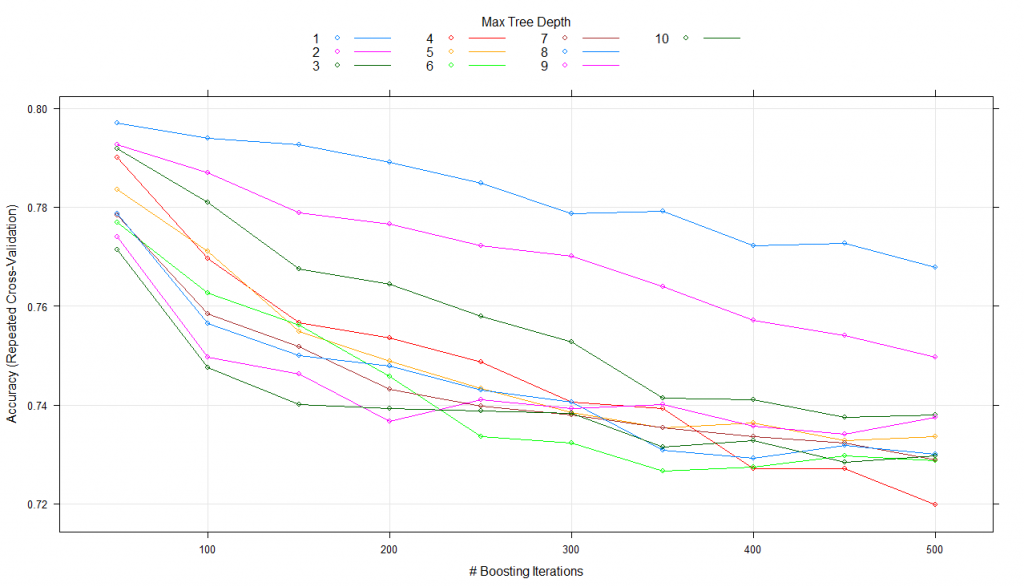Here, it keeps the shrinkage and n.minobsinnode parameters constant while alters n.trees and interaction.depth over 10 values and uses the best combination to train the final model with.

## 7. Variable importance estimation using caret

Caret also makes the variable importance estimates accessible with the use of varImp() for any model. Let’s have a look at the variable importance for all the four models that we created:

````#Checking variable importance for GBM`

`#Variable Importance`
`varImp(object=model_gbm)`
`#gbm variable importance`
`#Overall`
`#Credit_History    100.000`
`#LoanAmount         16.633`
`#ApplicantIncome     7.104`
`#CoapplicantIncome   6.773`
`#Loan_Amount_Term    0.000`

`#Plotting Varianle importance for GBM`
`plot(varImp(object=model_gbm),main="GBM - Variable Importance")````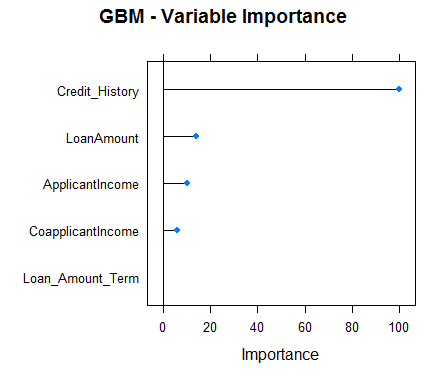````#Checking variable importance for RF`
`varImp(object=model_rf)`
`#rf variable importance`

`#Overall`
`#Credit_History     100.00`
`#ApplicantIncome     73.46`
`#LoanAmount          60.59`
`#CoapplicantIncome   40.43`
`#Loan_Amount_Term     0.00`

`#Plotting Varianle importance for Random Forest`
`plot(varImp(object=model_rf),main="RF - Variable Importance")````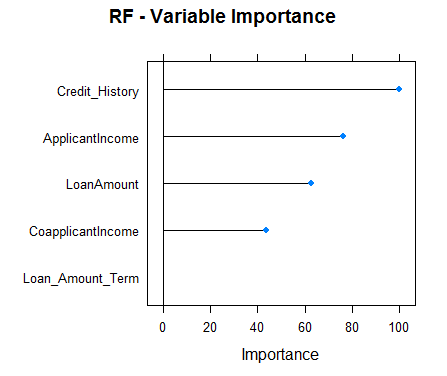````#Checking variable importance for NNET`
`varImp(object=model_nnet)`
`#nnet variable importance`

`#Overall`
`#ApplicantIncome    100.00`
`#LoanAmount          82.87`
`#CoapplicantIncome   56.92`
`#Credit_History      41.11`
`#Loan_Amount_Term     0.00`

`#Plotting Variable importance for Neural Network`
`plot(varImp(object=model_nnet),main="NNET - Variable Importance")````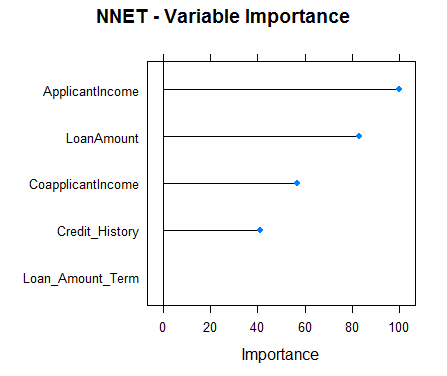````#Checking variable importance for GLM`
`varImp(object=model_glm)`
`#glm variable importance`

`#Overall`
`#Credit_History    100.000`
`#CoapplicantIncome  17.218`
`#Loan_Amount_Term   12.988`
`#LoanAmount          5.632`
`#ApplicantIncome     0.000`

`#Plotting Variable importance for GLM`
`plot(varImp(object=model_glm),main="GLM - Variable Importance")````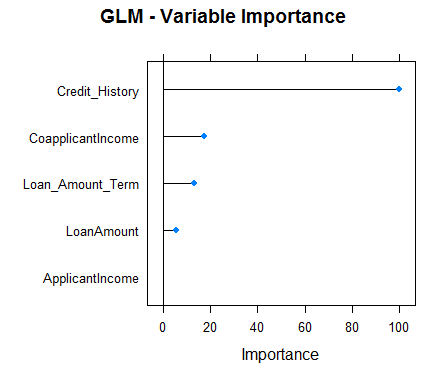Clearly, the variable importance estimates of different models differs and thus might be used to get a more holistic view of importance of each predictor. Two main uses of variable importance from various models are:

• Predictors that are important for the majority of models represents genuinely important predictors.
• Foe ensembling, we should use predictions from models that have significantly different variable importance as their predictions are also expected to be different. Although, one thing that must be make sure is that all of them are sufficiently accurate.

## 8. Predictions using Caret

For predicting the dependent variable for the testing set, Caret offers predict.train(). You need to specify the model name, testing data. For classification problems, Caret also offers another feature named type which can be set to either “prob” or “raw”. For type=”raw”, the predictions will just be the outcome classes for the testing data while for type=”prob”, it will give probabilities for the occurrence of each observation in various classes of the outcome variable.

Let’s take a look at the predictions from our GBM model:

````#Predictions`
`predictions<-predict.train(object=model_gbm,testSet[,predictors],type="raw")`
`table(predictions)`
`#predictions`
`#0   1`
`#28 125````

Caret also provides a confusionMatrix function which will give the confusion matrix along with various other metrics for your predictions. Here is the performance analysis of our GBM model:

````confusionMatrix(predictions,testSet[,outcomeName])`
`#Confusion Matrix and Statistics`
`#Reference`
`#Prediction   0   1`
`#0  25   3`
`#1  23 102`

`#Accuracy : 0.8301        `
`#95% CI : (0.761, 0.8859)`
`#No Information Rate : 0.6863        `
`#P-Value [Acc > NIR] : 4.049e-05     `
`#Kappa : 0.555         `
`#Mcnemar's Test P-Value : 0.0001944     `
`#Sensitivity : 0.5208        `
`#Specificity : 0.9714        `
`#Pos Pred Value : 0.8929`
`#Neg Pred Value : 0.8160        `
`#Prevalence : 0.3137        `
`#Detection Rate : 0.1634        `
`#Detection Prevalence : 0.1830        `
`#Balanced Accuracy : 0.7461        `
```#'Positive' Class : 0

``````

## End Notes

Caret is one of the most powerful and useful packages ever made in R. It alone has the capability to fulfill all the needs for predictive modeling from preprocessing to interpretation. Additionally, its syntax is also very easy to use. If you use R, I’ll encourage you to use Caret.

Caret is a very comprehensive package and instead of covering all the functionalities that it offers, I thought it’ll be a better idea to show an end-to-end implementation of Caret on a real hackathon J dataset. I have tried to cover as many functions in Caret as I could, but Caret has a lot more to offer. For going in depth, you might find the resources mentioned above very useful. Several of these resources have been written by Max Kuhn (the creator of caret package) himself.

### You can test your skills and knowledge. Check out Live Competitions and compete with best Data Scientists from all over the world.package in the comments below.

You can also read this article on Analytics Vidhya's Android APPThis article is quite old and you might not get a prompt response from the author. We request you to post this comment on Analytics Vidhya's Discussion portal to get your queries resolved

### 24 Comments

•KISHORE says:

Hello @Saurav Kaushik , I really appreciate you for sharing such a nice article on CART.
This really help a lot !

•Saurav Kaushik says:

Hey Kishore.

Glad you liked it. Happy to help!

•zhanyou Xu says:

where can I download the data used in this article? thank you.

zhanyou

•Miguel Hidalgo says:

Hi Saurav, how are you, nice article!. Saurav, where can I download the data file? train_u6lujuX_CVtuZ9i.csv

Thanks,

Miguel

•Hena Jose says:

Hi Saurav, Good article. Thank you very much for explaining the tuning function

•Saurav Kaushik says:

Hey, Hena jose. Happy it helped you!

•Canyanwu says:

Thank you Saurav. Assuming I have a new data-set (with same set of features), what line of code in python
allows me to make predictions (using your existing trained model)? I currently programme in MATLAB. Hope you can help with this. Thanks.

•Saurav Kaushik says:

Hey Canyanwu,

I don’t understand how you wish to make predictions using my model?
You first need to train the model on your machine and then go on to predict using that model.

•Prateek says:

Hi Saurav, while running this code

train_processed <- predict(preProcValues, train)

I am getting the following error:

Error in `[.data.frame`(old, , non_missing_cols, drop = FALSE) :
undefined columns selected

•Saurav Kaushik says:

Hey Pratik,

I re ran the code and didn’t ran into any error. I’ll suggest:

1. Make sure you have library RANN installed and loaded.
2. Make sure you have used preProcess() to create preProcValues like:

#Imputing missing values using KNN.Also centering and scaling numerical columns
preProcValues <- preProcess(train, method = c("knnImpute","center","scale"))

•Devin says:

I am getting an error on running the code line – train_processed <- predict(preProcValues, train)

Error in `[.data.frame`(old, , non_missing_cols, drop = FALSE) :
undefined columns selected

•Saurav Kaushik says:

Hey Devin,

I re ran the code and didn’t ran into any error. I’ll suggest:

1. Make sure you have library RANN installed and loaded.
2. Make sure you have used preProcess() to create preProcValues using:

#Imputing missing values using KNN.Also centering and scaling numerical columns
preProcValues <- preProcess(train, method = c("knnImpute","center","scale"))

•RamKasula32 says:

Thanks for Sharing

•AN1992 says:

Please explain the expand grid function
i am unable to understand its parameters, like shrinkage,, n.minobsinnode, n.minobsinnode
i am a new learner so do not have vast knowledge. Please explain, if their are any other parameters

•Saurav Kaushik says:

Hi,

These are the tunable parameters specific to the algorithm you are using. You can use the modelLookup() to get the tunable parameters for each mode as I have explained.

By using expand grid function you are simply asking to create the models with each possible combination of these parameters and get the one which gives the best cross validation performance.

Best,
Saurav.

•AN1992 says:

Thank You,
i would like to explore more, basically theoretical aspects of all machine learning algos.
i am really interested in this, i am not from CS background, i am a economist, had good grasp on statistical terms.
Help me

•hellozml says:

Thanks for the clear stepwise tutorial. 1st klass.

remember to add verbose=FALSE in the model train module to avoid screen endless status outputs…

•pengrunze says:

Thanks for Sharing.
I would like to know why you use one hot encoding in Caret to create dummy variables for each level of a categorical variable instead of using factor directly.

•Hector Alvaro Rojas says:

In the GLM model (logistic), how can I change the “‘Positive’ Class : 0” to “‘Positive’ Class : 1”?

In my dataset I have a variable “Tclient” with two values: Bad; Good.

Using MLR R library I define the Positive Class as:

trainTask <- makeClassifTask(data = train,target = "Tclient", positive = "Good")

How can I do this using Caret?

•Behrouz says:

Thanks a million! I learnt a lot from it.

•Narendhar says:

Hiii Sourav when I run this iam getting this error please help me.

Medicin_sales = rfe(trainSet[,predictors], trainSet[,outcomeName],
+ rfeControl = control)
Error in `[.data.frame`(trainSet, , outcomeName) :
undefined columns selected

•Aishwarya Singh says:

Hi Narendhra,

The code is working fine for me. Please make sure you have followed the steps as in the article. If you still face any issue, do let us know.Download ResourceDownload Resource